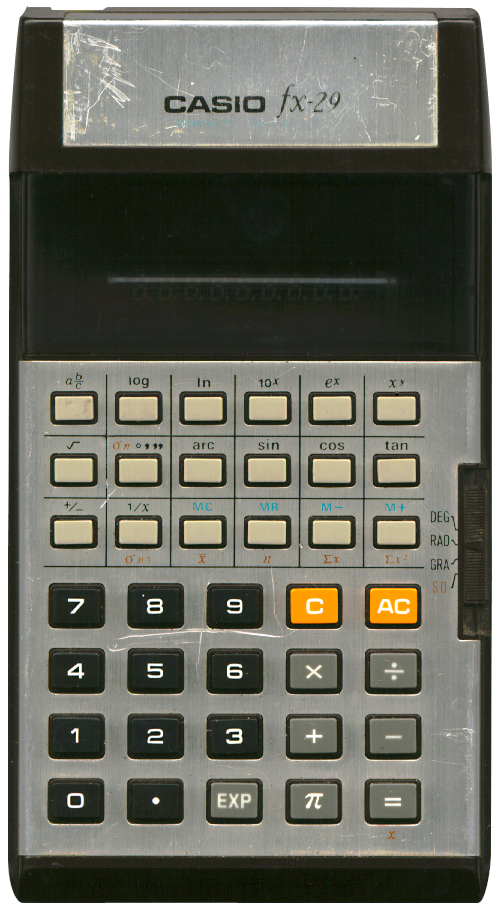# CASIO fx‑29

## Description

 Brand: CASIO Model: fx-29 Type: Scientific calculator Picture:Batteries: AA x 2 Lifetime: Introduced: 1977 Terminated: unknown Notes: A CASIO scientific calculator with a fluorescent display, a slider to switch between modes, and capable of calculating with fractions and statistical operations. Quite similar to the Casio fx-19. As far as I can tell the main difference is the layout, the casing and the number of batteries. The workings of the 'xy'-key might be puzzling to users of this calculator. When you enter a (positive) number and press the 'xy'-key an intermediate result is shown, being the natural log 'ln' of the number entered. To obtain its power enter the value for y and press the '='-key. My guess is that this calculator uses the formula xy = e(y · ln x). This works nicely for positive values of x but will result in an error when x is zero or less. Not a big problem because powers of negavive numbers behave counter intuitive anyway (producing non-complex results only for integer values of y or fractional values of y with an odd denominator). This calculator has separate keys for the exponent and the constant π instead of the combined key of most other scientific calculators by CASIO (where the "EXP"-key will enable you to enter the numbers exponent when you first enter digits, and will otherwise enter the constant π). There's also no "INV"-key, only an "arc"-key for the inverse trigonometric functions. Statistical functions are available when the mode selector slider is all the way to the bottom. Mine is quite worn out but still working fine, although one needs to press hard down on its buttons sometimes.